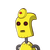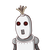# THE MEASURE OF THE SIDE OF A SQUARE LAWN OF AREA 1849m2​

THE MEASURE

OF THE SIDE

OF A SQUARE

LAWN OF

AREA 1849m2​

### 2 thoughts on “THE MEASURE <br /><br />OF THE SIDE <br /><br />OF A SQUARE <br /><br />LAWN OF <br /><br />AREA 1849m2​”

1.Step-by-step explanation:

THE AREA OF THE SQUARE = 1849m²

THE LENGTH OF THE SQUARE

=√1849 m

=√43×43 m

= 43m

THE PERIMETER OF THE SQUARE

=4×43m

=172m

ANSWER) THE LENGTH OF THE SQUARE IS 43m AND THE PERIMETER IS 172m.

HERE YOU GO.

2.